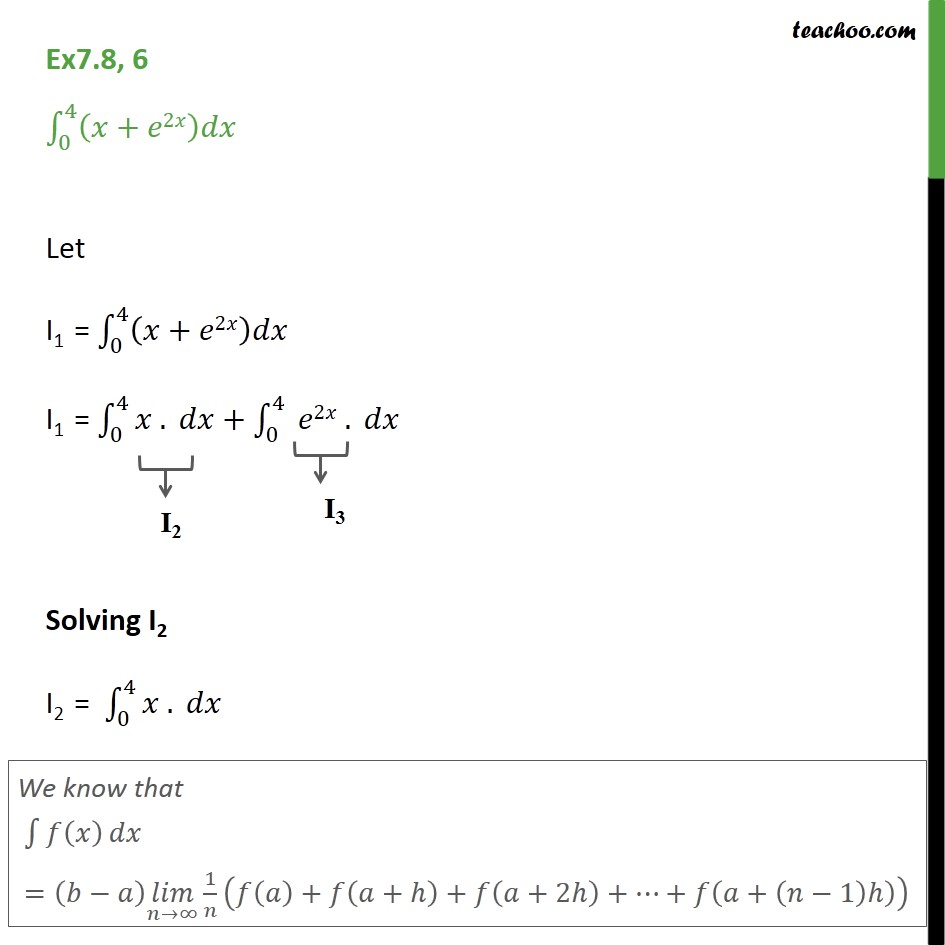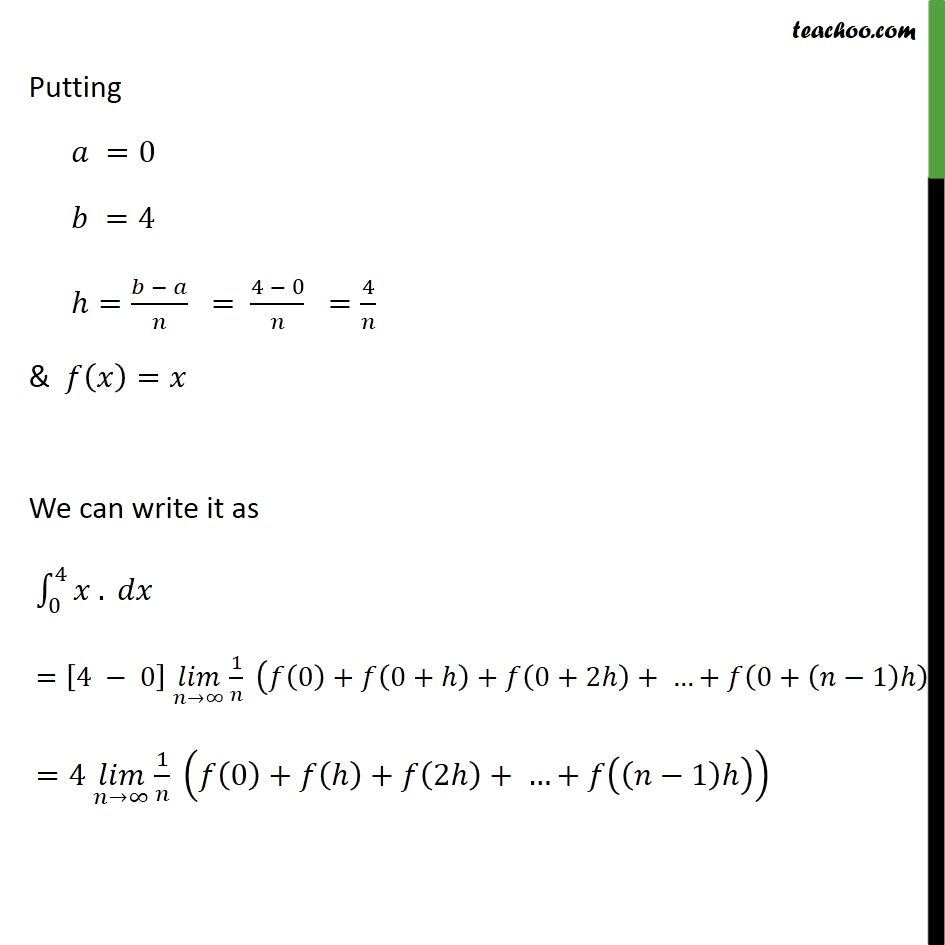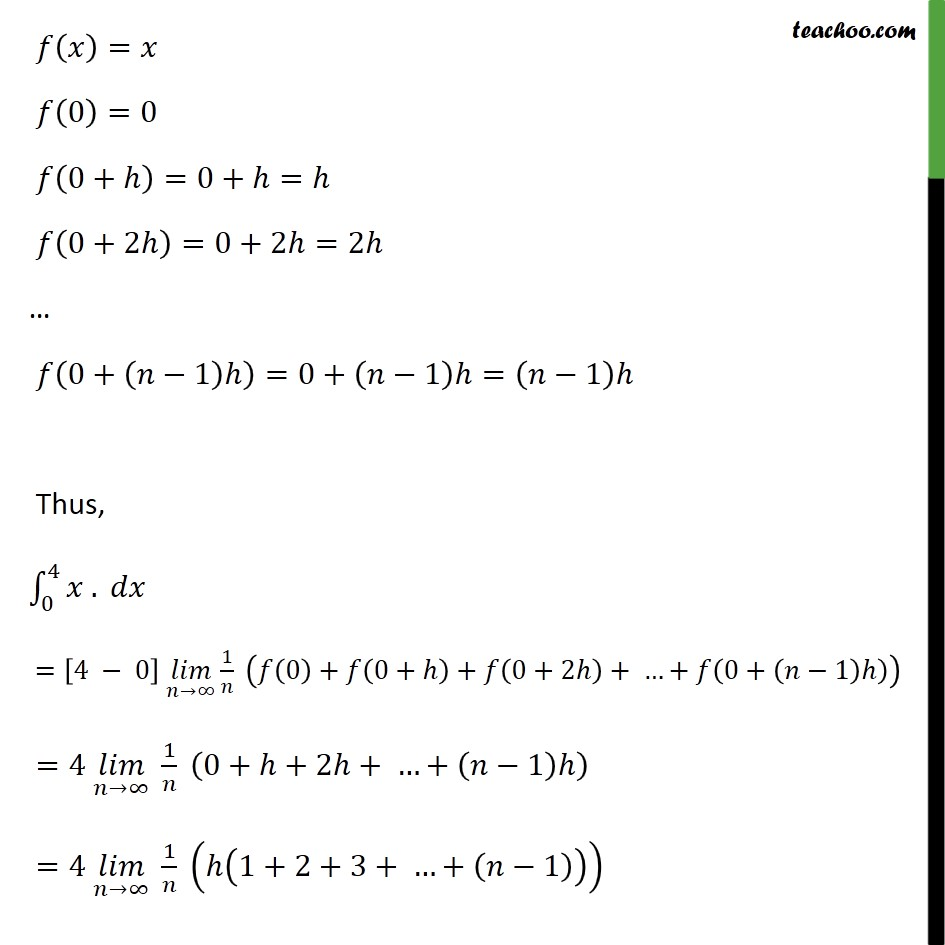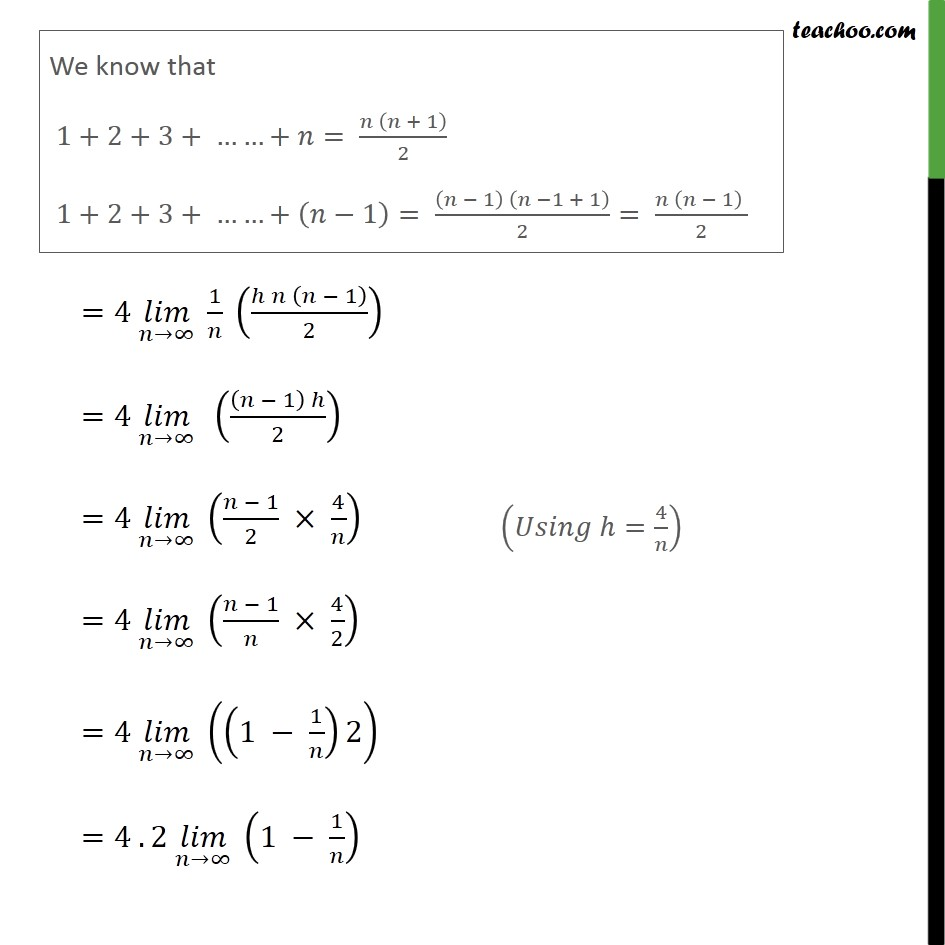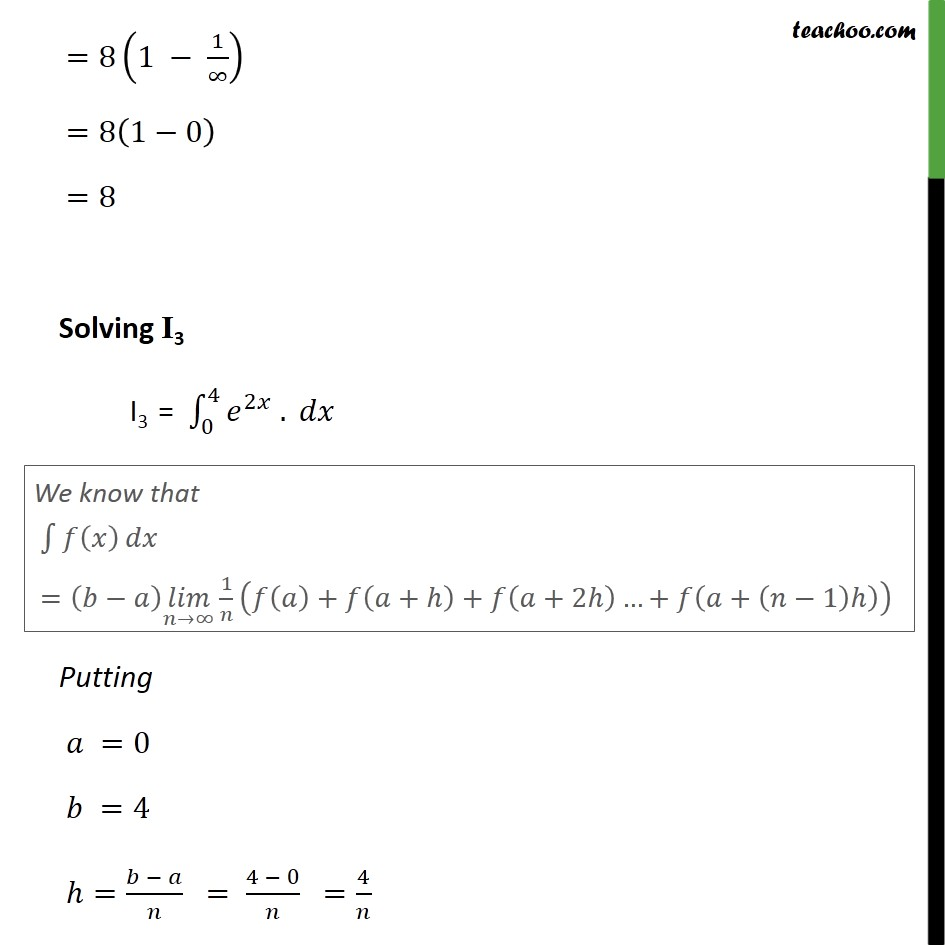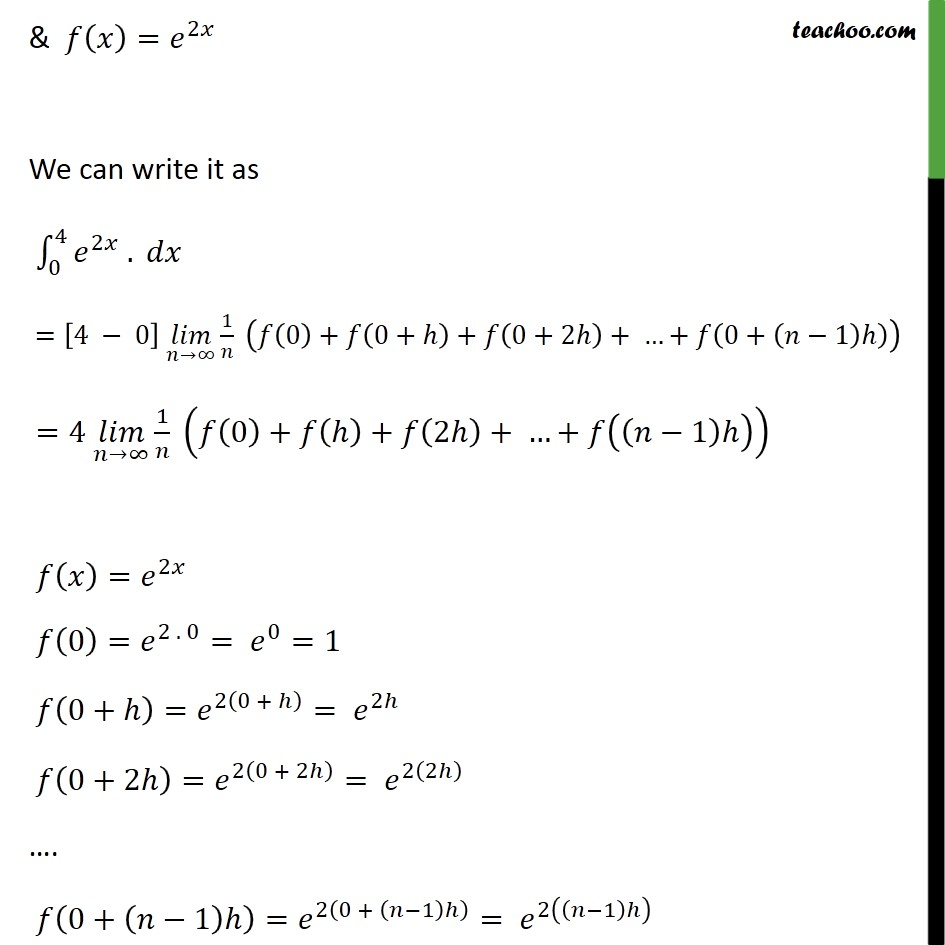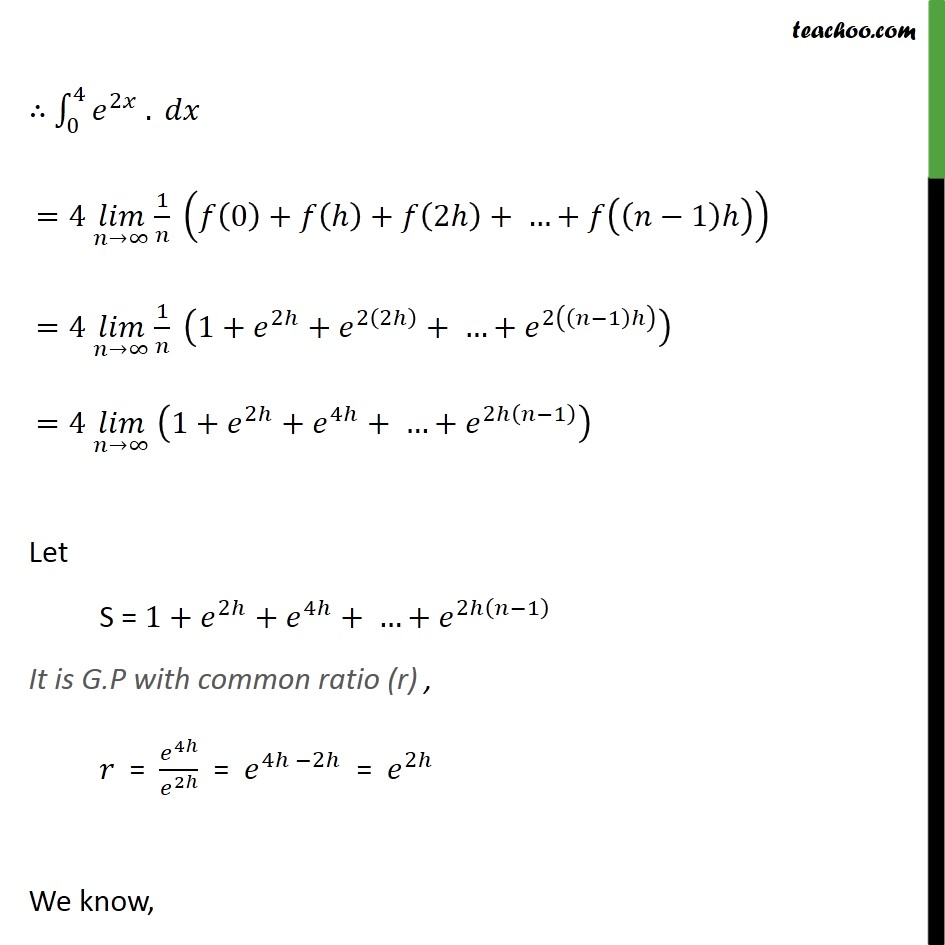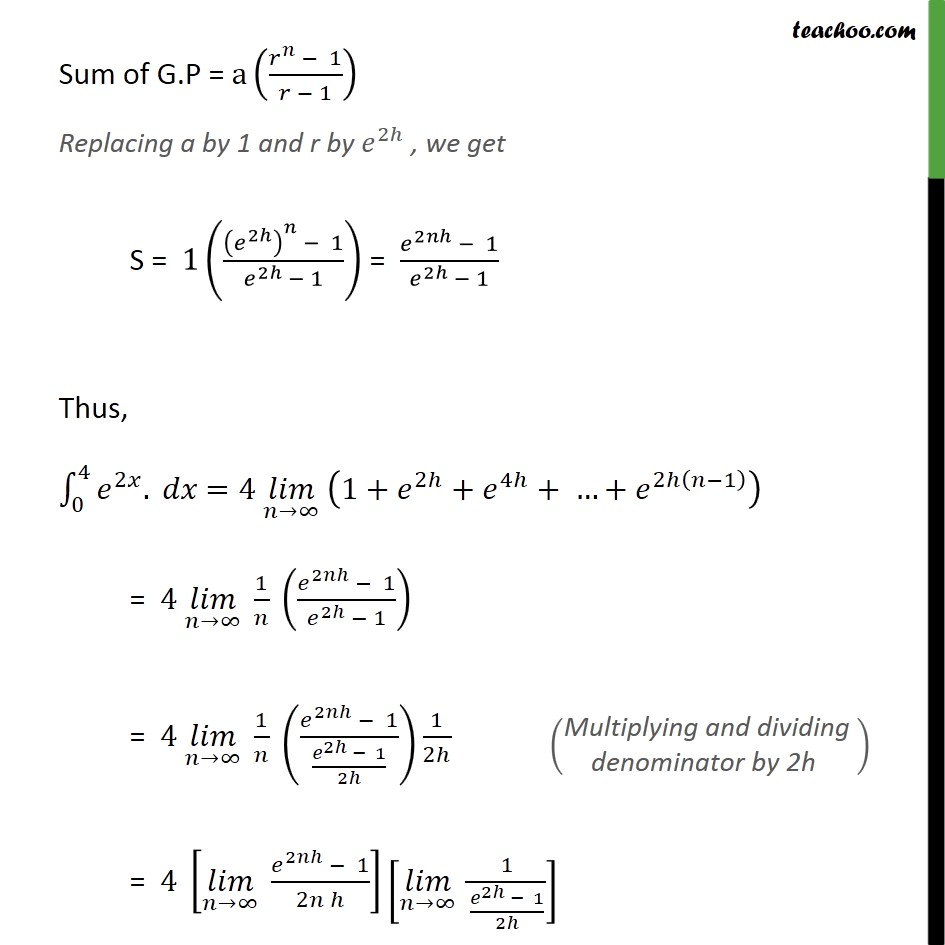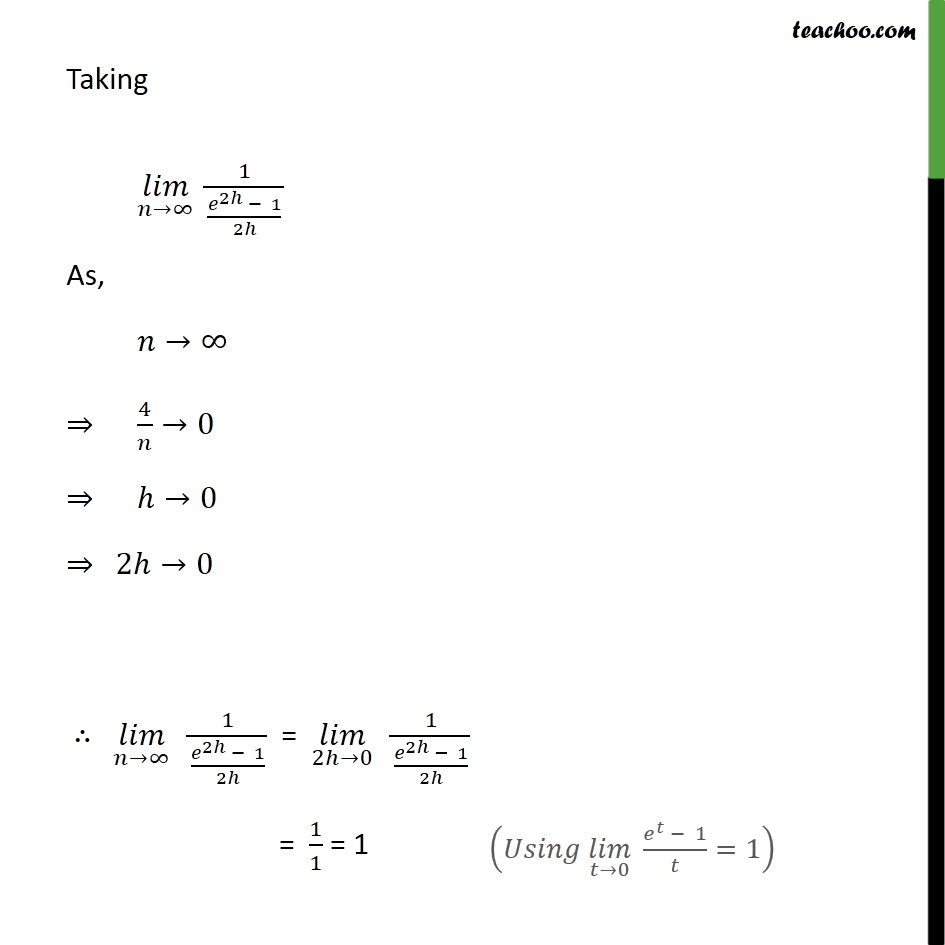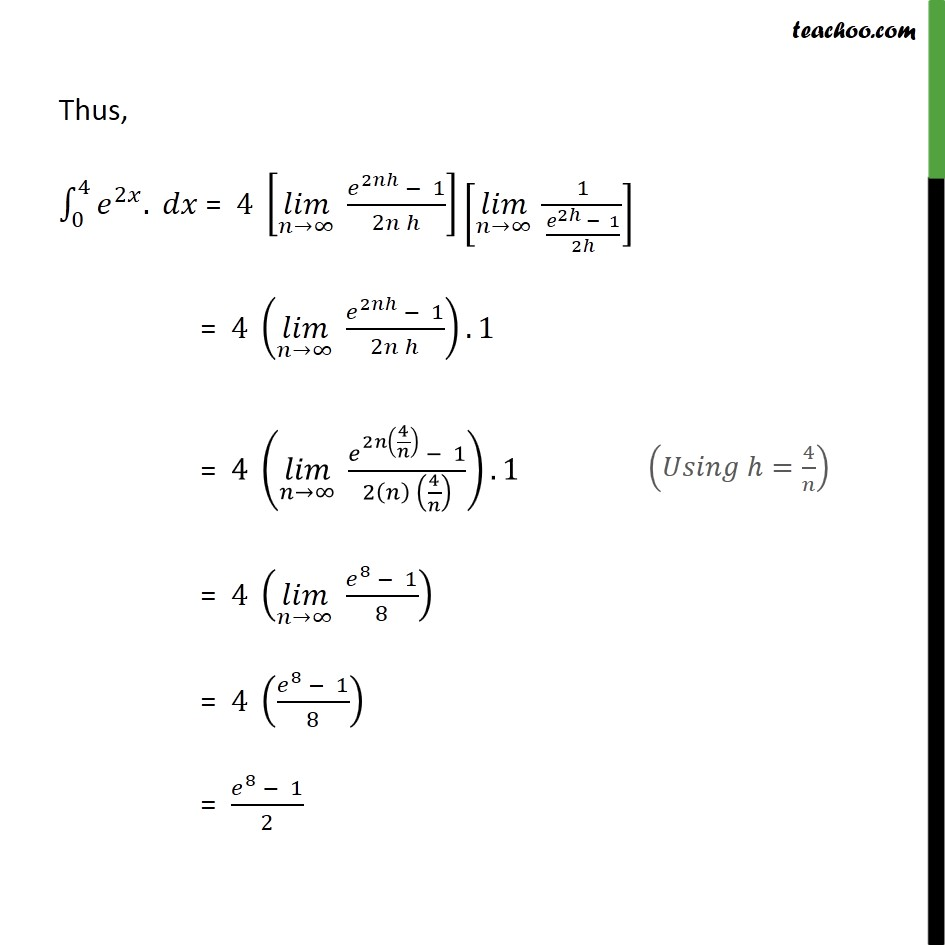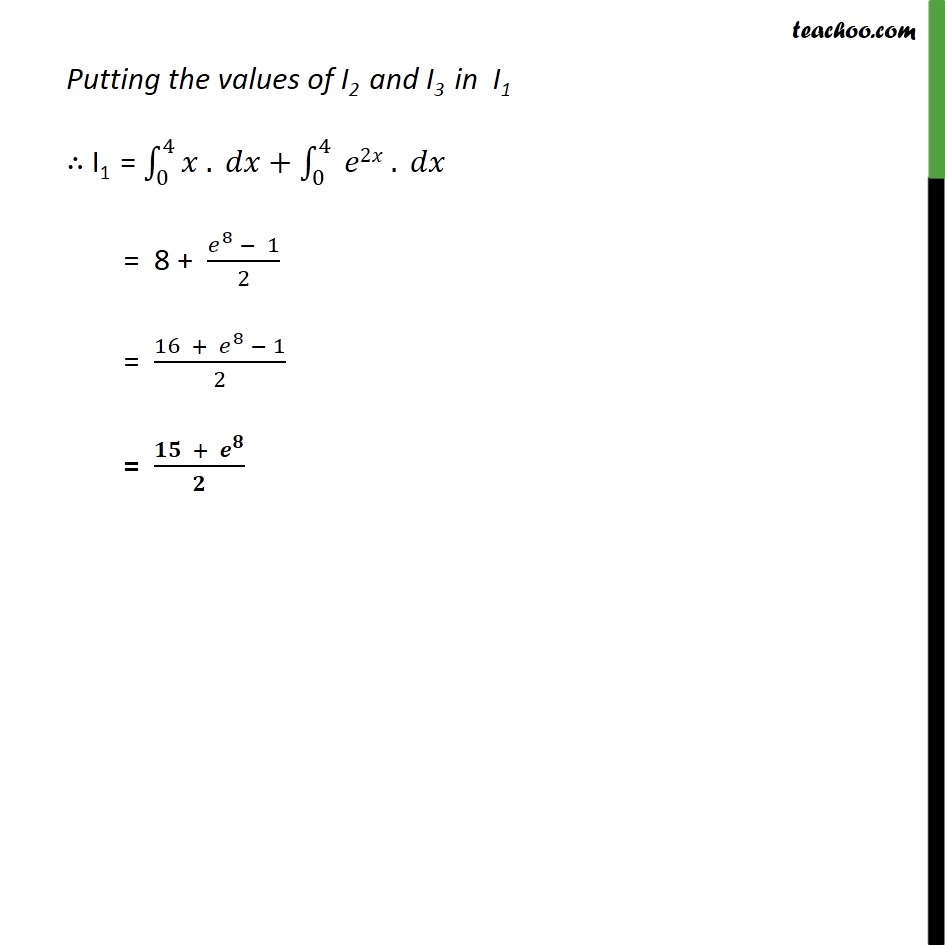1. Chapter 7 Class 12 Integrals
2. Serial order wise
3. Ex 7.8

Transcript

Ex7.8, 6 0 4 + 2 Let I1 = 0 4 + 2 I1 = 0 4 . + 0 4 2 . Solving I2 I2 = 0 4 . Putting =0 =4 = = 4 0 = 4 & = We can write it as 0 4 . = 4 0 uc1 1 0 + 0+ + 0+2 + + 0+ 1 =4 uc1 1 0 + + 2 + + 1 = 0 =0 0+ =0+ = 0+2 =0+2 =2 0+ 1 =0+ 1 = 1 Thus, 0 4 . = 4 0 uc1 1 0 + 0+ + 0+2 + + 0+ 1 =4 uc1 1 0+ +2 + + 1 =4 uc1 1 1+2+3+ + 1 =4 uc1 1 1 2 =4 uc1 1 2 =4 uc1 1 2 4 =4 uc1 1 4 2 =4 uc1 1 1 2 =4 . 2 uc1 1 1 =8 1 1 uc1 =8 1 0 =8 Solving I3 I3 = 0 4 2 . Putting =0 =4 = = 4 0 = 4 & = 2 We can write it as 0 4 2 . = 4 0 uc1 1 0 + 0+ + 0+2 + + 0+ 1 =4 uc1 1 0 + + 2 + + 1 = 2 0 = 2 . 0 = 0 =1 0+ = 2 0 + = 2 0+2 = 2 0 + 2 = 2 2 . 0+ 1 = 2 0 + 1 = 2 1 0 4 2 . =4 uc1 1 0 + + 2 + + 1 =4 uc1 1 1+ 2 + 2 2 + + 2 1 =4 uc1 1+ 2 + 4 + + 2 1 Let S = 1+ 2 + 4 + + 2 1 It is G.P with common ratio (r) , = 4 2 = 4 2 = 2 We know, Sum of G.P = a 1 1 Replacing a by 1 and r by 2 , we get S = 1 2 1 2 1 = 2 1 2 1 Thus, 0 4 2 . =4 uc1 1+ 2 + 4 + + 2 1 = 4 uc1 1 2 1 2 1 = 4 uc1 1 2 1 2 1 2 1 2 = 4 uc1 2 1 2 uc1 1 2 1 2 Taking uc1 1 2 1 2 As, 4 0 0 2 0 uc1 1 2 1 2 = 2 0 1 2 1 2 = 1 1 = 1 Thus, 0 4 2 . = 4 uc1 2 1 2 uc1 1 2 1 2 = 4 uc1 2 1 2 .1 = 4 uc1 2 4 1 2 4 .1 = 4 uc1 8 1 8 = 4 8 1 8 = 8 1 2 Putting the values of I2 and I3 in I1 I1 = 0 4 . + 0 4 2 . = 8 + 8 1 2 = 16 + 8 1 2 = +

Ex 7.8

Chapter 7 Class 12 Integrals
Serial order wise